Courses
Courses for Kids
Free study material
Free LIVE classes
More

# NCERT Solutions for Class 9 Maths Chapter 7 - ExerciseLIVE
Join Vedantu’s FREE Mastercalss

## NCERT Solutions for Class 9 Maths Chapter 7 Triangles (Ex 7.5) Exercise 7.5

Free PDF download of NCERT Solutions for Class 9 Maths Chapter 7 Exercise 7.5 (Ex 7.5) and all chapter exercises at one place prepared by expert teacher as per NCERT (CBSE) books guidelines. Class 9 Maths Chapter 7 Triangles Exercise 7.5 Questions with Solutions to help you to revise complete Syllabus and Score More marks. Register and get all exercise solutions in your emails.

Last updated date: 22nd Sep 2023
Total views: 512.1k
Views today: 13.12k
Watch videos on
NCERT Solutions for Class 9 Maths Chapter 7 - Exercise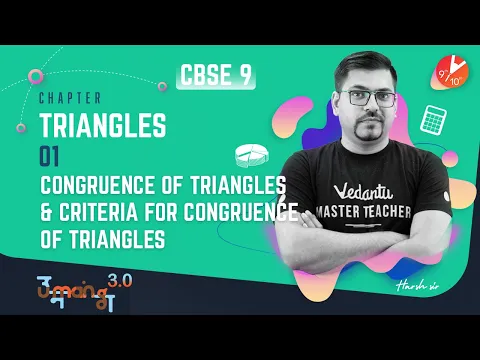TRIANGLES L-1 (𝐂𝐨𝐧𝐠𝐫𝐮𝐞𝐧𝐜𝐞 𝐨𝐟 𝐓𝐫𝐢𝐚𝐧𝐠𝐥𝐞𝐬 & 𝐂𝐫𝐢𝐭𝐞𝐫𝐢𝐚 𝐟𝐨𝐫 𝐂𝐨𝐧𝐠𝐫𝐮𝐞𝐧𝐜𝐞 𝐨𝐟 𝐓𝐫𝐢𝐚𝐧𝐠𝐥𝐞𝐬) CBSE 9 Maths -Term 1
Vedantu 9&10
SubscribeShare
3.1K likes
89.3K Views
2 years ago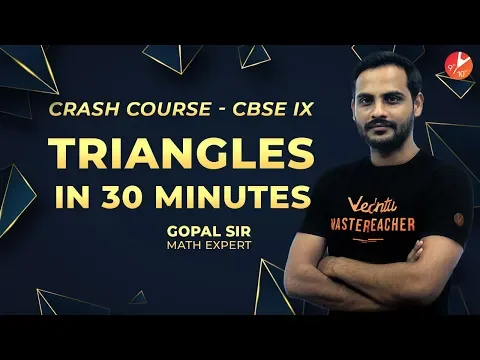Triangles in 30 Minutes | Crash Course | Maths | Gopal Sir | Vedantu Class 9
Vedantu 9&10
10.4K likes
300.1K Views
4 years ago
Play Quiz

## Access NCERT Solutions for Class 9 Mathematics Chapter 7 - Triangles

Exercise 7.5

1. ABC is a triangle. Locate a point in the interior of ΔABC which is equidistant from all the vertices of ΔABC.

Ans: The circumcentre of a triangle is always equidistant from all of its   vertices.

The circumcentre is the point where the perpendicular bisectors of all the triangle's sides meet.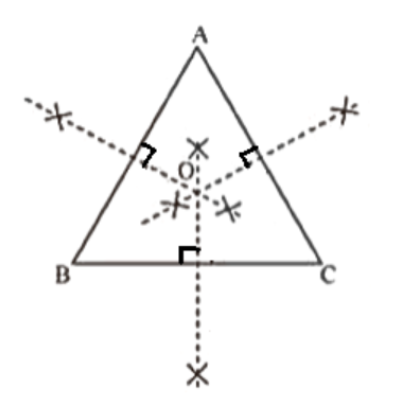By sketching the perpendicular bisectors of the triangle's sides AB, BC, and CA, we may determine the circumcentre in\$\Delta ABC\$. The intersection of these bisectors is marked by the letter O. As a result, O is the point that is equidistant from all of \$\Delta ABC\$'s vertices.

2. In a triangle locate a point in its interior which is equidistant from all the sides of the triangle.

Ans: The incentre of a triangle is the point that is equidistant from all the triangle's sides. The intersection point of the angle bisectors of a triangle's interior angles is the centre of the triangle.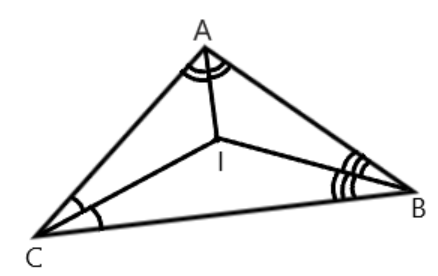By drawing the angle bisectors of the interior angles of this triangle in \$\Delta ABC\$, we may get the incentive of this triangle. It is the point at which these angle bisectors cross each other. As a result, it is the point that is equidistant from all of \$\Delta ABC\$'s sides.

3. In a huge park people are concentrated at three points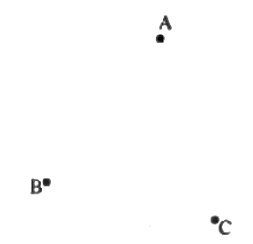A. where there are different slides and swings for children,

B. near which a man-made lake is situated,

C. which is near to a large parking and exit.

Where should an ice-cream parlour be set up so that the maximum number of persons can approach it?

(Hint: The parlor should be equidistant from A, B and C)

Ans: If the ice cream parlour is equidistant from A, B, and C, the maximum number of people can approach it. A, B, and C now form a triangle. The circumcentre is the only point in a triangle that is equidistant from its vertices. As a result, the ice cream parlour should be located near ΔABC's circumcenter O.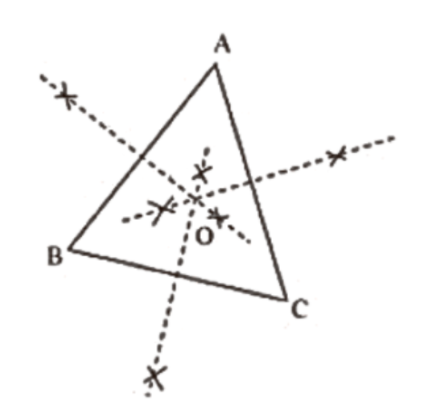The maximum number of people can approach it in this case.

By drawing perpendicular bisectors of the sides of this triangle, we can find the circumcentre O.

4. Complete the hexagonal and star shaped Rangolies by filling them with as many equilateral triangles of side 1 cm as you can. Count the number of triangles in each case. Which has more triangles?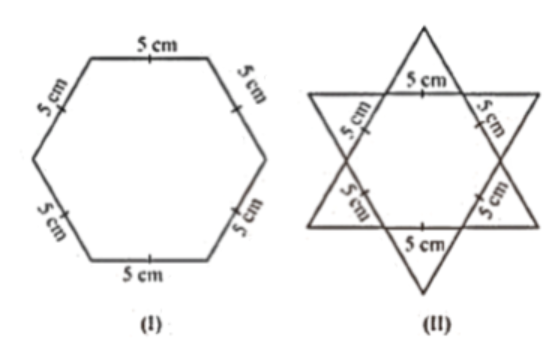Ans: We can see that the hexagonal shaped rangoli has a total of 6 equilateral triangles of side 5 cm in it.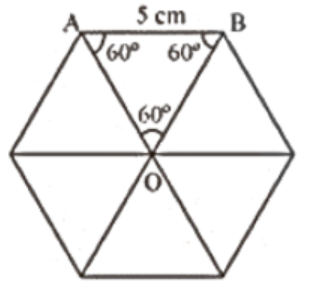The star-shaped rangoli also has 6 equilateral triangles of side 5cm and a hexagon in the middle.

Therefore, star-shaped rangoli will be filled with more equilateral triangles of side 1 cm in it.

## NCERT Solutions for Class 9 Maths Chapter 7 Triangles

Opting for the NCERT solutions for Ex 7.5 Class 9 Maths is considered as the best option for the CBSE students when it comes to exam preparation. This chapter consists of many exercises. Out of which we have provided the Exercise 7.5 Class 9 Maths NCERT solutions on this page in PDF format. You can download this solution as per your convenience or you can study it directly from our website/ app online.

Vedantu in-house subject matter experts have solved the problems/ questions from the exercise with the utmost care and by following all the guidelines by CBSE. Class 9 students who are thorough with all the concepts from the Subject Triangles textbook and quite well-versed with all the problems from the exercises given in it, then any student can easily score the highest possible marks in the final exam. With the help of this Class 9 Maths Chapter 7 Exercise 7.5 solutions, students can easily understand the pattern of questions that can be asked in the exam from this chapter and also learn the marks weightage of the chapter. So that they can prepare themselves accordingly for the final exam.

Besides these NCERT solutions for Class 9 Maths Chapter 7 Exercise 7.5, there are plenty of exercises in this chapter which contain innumerable questions as well. All these questions are solved/answered by our in-house subject experts as mentioned earlier. Hence all of these are bound to be of superior quality and anyone can refer to these during the time of exam preparation. In order to score the best possible marks in the class, it is really important to understand all the concepts of the textbooks and solve the problems from the exercises given next to it.

Do not delay any more. Download the NCERT solutions for Class 9 Maths Chapter 7 Exercise 7.5 from Vedantu website now for better exam preparation. If you have the Vedantu app in your phone, you can download the same through the app as well. The best part of these solutions is these can be accessed both online and offline as well.

## FAQs on NCERT Solutions for Class 9 Maths Chapter 7 - Exercise

1. What are the properties of an isosceles triangle, according to Chapter 7 of Class 9 Maths?

In an isosceles triangle, the two sides of a triangle are equal, and the angles opposite to the equal sides are also equal. To get more answers related to the exercises in this chapter, visit Vedantu and get all the explained answers for Chapter 7 of Class 9 Maths in one place. Practicing these will give students a good idea of how the questions should be solved from an exam point of view.

2. Can students solve Exercise 7.5 of Chapter 7 of Class 9 Maths in two days?

Yes, students can solve the entire Chapter 7 of Class 9 Maths exercises within two days. All they need is a good hold over the basics of the chapter. The best place to get the basics stronger is Vedantu. Students can get step-to-step answers for every question in all the exercises of the Chapter 7 of Class 9 Maths book. These answers have been brought to you by experts who give their best to make solutions as simple as possible.

3. Why should we do the optional Exercise 7.5 of Chapter 7 of Class 9 Maths?

You should solve the optional Exercise 7.5 of Chapter 7 of Class 9 Maths because it helps strengthen your basics in triangles' properties, especially in the equalities and inequalities in a triangle. This exercise is not so important from an exam viewpoint. However, it can add to your knowledge base and help you get a much clearer understanding of what you have been studying.

4. What is an SAS congruent triangle, according to Chapter 7 of Class 9 Maths?

If the two sides and one included angle of a triangle are equal to the two sides, and one included angle of the other triangle. Then we can say that the two triangles meet the criteria for SAS (side-angle-side) congruency. You will get full NCERT Solutions Class 9 Maths Chapter 7 on Vedantu. The explanations to sums have been provided by experts who know how students can score well in their examinations.

5. Is Chapter 7 of Class 9 Maths very tough?

Maths is a subject that seems tough to students, but with rigorous practice, students can easily get command over Chapter 7 of Class 9 Maths. So, considering Maths a difficult subject is a matter of mindset. If you practice Chapter 7 of Class 9 Maths daily, you will not lag and will understand everything that is being taught. If you get stuck anywhere, you can easily check out the NCERT Solutions for Chapter 7 of Class 9 Maths available at free of cost on the Vedantu website and on the Vedantu app for any clarifications.